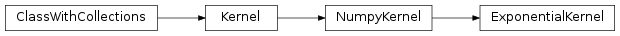# mvpa2.measures.irelief.ExponentialKernel¶class `mvpa2.measures.irelief.``ExponentialKernel`(*args, **kwargs)

The Exponential kernel class.

Note that it can handle a length scale for each dimension for Automtic Relevance Determination.

Attributes

 `descr` Description of the object if any

Methods

 `add_conversion`(typename, methodfull, methodraw) Adds methods to the Kernel class for new conversions `as_ls`(kernel) `as_np`() Converts this kernel to a Numpy-based representation `as_raw_ls`(kernel) `as_raw_np`() Directly return this kernel as a numpy array. `as_raw_sg`(kernel) Converts directly to a Shogun kernel `as_sg`(kernel) Converts this kernel to a Shogun-based representation `cleanup`() Wipe out internal representation `compute`(ds1[, ds2]) Generic computation of any kernel `compute_lml_gradient`(alphaalphaT_Kinv, data) Compute grandient of the kernel and return the portion of log marginal likelihood gradient due to the kernel. `compute_lml_gradient_logscale`(...) Compute grandient of the kernel and return the portion of log marginal likelihood gradient due to the kernel. `computed`(\*args, \*\*kwargs) Compute kernel and return self `gradient`(data1, data2) Compute gradient of the kernel matrix. `reset`()

Initialize instance of ExponentialKernel

Parameters: length_scale : float or list(float), optional The characteristic length-scale (or length-scales) of the phenomenon under investigation. Constraints: value must be convertible to type ‘float’, or value must be convertible to list(float). [Default: 1.0] sigma_f : float, optional Signal standard deviation. Constraints: value must be convertible to type ‘float’. [Default: 1.0] enable_ca : None or list of str Names of the conditional attributes which should be enabled in addition to the default ones disable_ca : None or list of str Names of the conditional attributes which should be disabled

Attributes

 `descr` Description of the object if any

Methods

 `add_conversion`(typename, methodfull, methodraw) Adds methods to the Kernel class for new conversions `as_ls`(kernel) `as_np`() Converts this kernel to a Numpy-based representation `as_raw_ls`(kernel) `as_raw_np`() Directly return this kernel as a numpy array. `as_raw_sg`(kernel) Converts directly to a Shogun kernel `as_sg`(kernel) Converts this kernel to a Shogun-based representation `cleanup`() Wipe out internal representation `compute`(ds1[, ds2]) Generic computation of any kernel `compute_lml_gradient`(alphaalphaT_Kinv, data) Compute grandient of the kernel and return the portion of log marginal likelihood gradient due to the kernel. `compute_lml_gradient_logscale`(...) Compute grandient of the kernel and return the portion of log marginal likelihood gradient due to the kernel. `computed`(\*args, \*\*kwargs) Compute kernel and return self `gradient`(data1, data2) Compute gradient of the kernel matrix. `reset`()
`compute_lml_gradient`(alphaalphaT_Kinv, data)

Compute grandient of the kernel and return the portion of log marginal likelihood gradient due to the kernel. Shorter formula. Allows vector of lengthscales (ARD) BUT THIS LAST OPTION SEEMS NOT TO WORK FOR (CURRENTLY) UNKNOWN REASONS.

`compute_lml_gradient_logscale`(alphaalphaT_Kinv, data)

Compute grandient of the kernel and return the portion of log marginal likelihood gradient due to the kernel. Shorter formula. Allows vector of lengthscales (ARD). BUT THIS LAST OPTION SEEMS NOT TO WORK FOR (CURRENTLY) UNKNOWN REASONS.

`gradient`(data1, data2)

Compute gradient of the kernel matrix. A must for fast model selection with high-dimensional data.